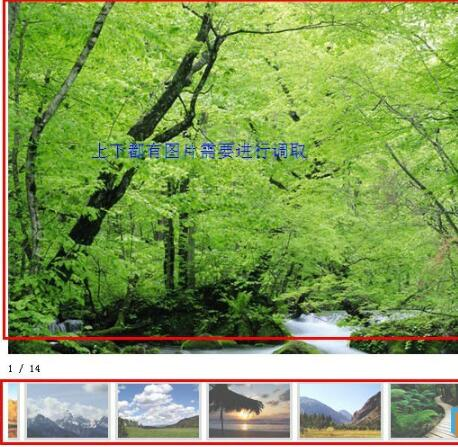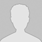>> 更多

### 织梦模板dedecms获取图片集多张图片及注释的教程`01` `1、在/include/common.func.php 里加上方法 //循环输出多张图片``function`
 `02` `function` `Getimgs(\$aid, \$imgwith = 300, \$imgheight = 270, \$num = 0, \$style = ``''``){`
 `03` `    ``global \$dsql;`
 `04` `    ``\$imgurls = ``''``;`
 `05` `     ``\$row = \$dsql -> getone(``"Select imgurls From`dede_addonimages` where aid='\$aid'"``); //`
 `06` `     ``\$imgurls = \$row[``'imgurls'``];`
 `07` `     ``preg_match_all(``"/{dede:img (.*)}(.*){/dede:img/isU"``, \$imgurls, \$wordcount);`
 `08` `     ``\$count = count(\$wordcount);`
 `09` `     ``if` `(\$num > \$count || \$num == 0){`
 `10` `        ``\$num = \$count;`
 `11` `    ``}`
 `12` `    `
 `13` `    ``for``(\$i = 0;\$i < \$num;\$i++){`
 `14` `        ``if``(\$style == ``'li'``){`
 `15` `            ``\$imglist .= ``"
• "``;`
 `16` `        ``}``else``{`
 `17` `            ``\$imglist .= ``""``;`
 `18` `        ``}`
 `19` `    ``}`
 `20` `     ``return` `\$imglist;`
 `21` `     ``}`

2、在模板里调用方法{dede:field.id function="Getimgs(@me,400,400,10)" /}

~~~~~~~~~~~~~~~~~~~~~~~~~~~~~~~~~~~~~~~~~~~~~~~~~~~~~~~~~~~~

注释:<img src= " . trim(\$wordcount[\$i]) . " width=" . \$imgwith . "height=" . \$imgheight . ">"是调取出来的格式，可根据自己需要的样式进行定义，比如加个li或者 添加class等等，这里就不多讲了。

希望这篇文章对大家有帮助，如果有错误，欢迎大家多多定评指出噢。交流才会进步！

以下方法是调取图片集多张图片注释的方法，操作类似。

1，在/include/common.func.php 里加上

//循环输出多张图片注释

 `01` `function` `Getimgnote(\$aid, \$num = 0){`
 `02` `    ``global \$dsql;`
 `03` `    ``\$imgurls = ``''``;`
 `04` `     ``\$row = \$dsql -> getone(``"Select imgurls ` where aid='\$aid'"``); //`
 `05` `     ``\$imgurls = \$row[``'imgurls'``];`
 `06` `     ``preg_match_all(``"|text='(.*)' width|U"``, \$imgurls, \$wordcount);`
 `07` `     ``\$count = count(\$wordcount);`
 `08` `     ``if` `(\$num > \$count || \$num == 0){`
 `09` `        ``\$num = \$count;`
 `10` `    ``}`
 `11` `    ``for``(\$i = 0;\$i < \$num;\$i++){`
 `12` `        ``if``(\$style == ``'li'``){`
 `13` `            ``\$imglist .= ``"
• "``;`
 `14` `        ``}``else``{`
 `15` `            ``\$imglist .= ``"
"``. trim(\$wordcount[\$i]) .``"
"``;`
 `16` `        ``}`
 `17` `    ``}`
 `18` `     ``return` `\$imglist;`
 `19` `     ``}`

2、在模板里调用方法{dede:field.id function="Getimgnote(@me,10)" /}说点什么吧
• 全部评论（0
还没有评论，快来抢沙发吧！